# Best Python Libraries for Data Science in 2024Python is a high-level programming language widely used for web dev, data science, AI, and scientific computing. It is known for its simple syntax which enables new users to quickly adapt to it. It also has a large and active developer community that has contributed many libraries and frameworks for tasks like machine learning, natural language processing, and image manipulation. Python, which has a strong emphasis on code readability and maintainability, can be used for small and large-scale projects. In this article, we will discuss the best Python libraries for data science that you can learn in 2024.

## Why Python?

Python is a popular choice for data science and data analytics due to several reasons:

1. It has a large community of users, which means that there is a wealth of resources available online, such as documentation, tutorials, and libraries.

2. Python has several powerful libraries for data manipulation and analysis. These include NumPy, Pandas, and scikit-learn which make it easy to perform complex tasks like linear algebra, statistical modeling, and machine learning.

3. Being a high-level language means that it is easy to read, write, and understand. This is important in data science where code readability and maintainability are key.

4. Python is highly extensible, which means that it can be easily integrated with other languages and tools. This is also important in data science where you often need to use a range of technologies to solve problems.

### NumPy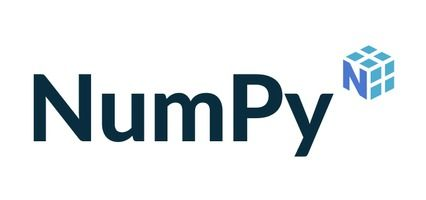NumPy is a staple library for scientific and analytics-related computing in Python. It consists of multi-dimensional arrays and matrices as well as in-built functions to perform mathematical transformations and operations on the same. NumPy is particularly useful for performing linear algebra operations, such as solving linear equations and performing matrix multiplications.

Some of the key features include:

• ndarray (n-dimensional array) object: This is a flexible array object that allows you to store and manipulate large collections of homogeneous data (data of the same type like integers or floating point values).
• Mathematical functions: It provides mathematical functions like trigonometric functions, exponential and logarithmic functions, and statistical functions.
• Linear algebra functions: It has functions for performing linear algebra operations. These can comprise matrix multiplications, solving linear equations, and finding the inverse of a matrix.
• Random number generation: NumPy has functions for generating random numbers from various probability distributions.
• Array manipulation functions: It also provides functions for reshaping, sorting, and manipulating the data in arrays.

### Pandas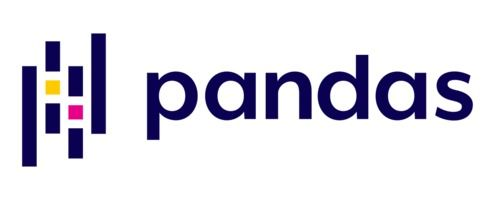Pandas is a library for data manipulation and analysis. It provides data structures for efficiently storing and manipulating large amounts of tabular data and tools for working with time series data. It also has a wide range of functions for reading and writing data to and from various file formats like CSV, Excel, and SQL databases.

This is arguably the most useful data science module out there. Here are some of the key features and characteristics:

• Data structures: Pandas provides two main data structures for storing and manipulating data: Series and DataFrame.
• Series: A Series is a one-dimensional array-type object that can contain any type of data. It is identical to a column in a spreadsheet or a field in a database table.
• DataFrame: A DataFrame is a two-dimensional table of data with rows and columns. It is analogous to a Microsoft spreadsheet or a database table.
• Indexing: There are a variety of ways to index and slice data, including label-based indexing (using row and column labels) and position-based indexing (using integer indices).
• Handling missing data: There are functions for handling missing data, such as filling missing values with a specified value or dropping rows or columns with missing data.
• Merging and joining: There are also functions for merging and joining data from different sources like databases, CSV files, and Excel sheets.
• Grouping and aggregation: Pandas offers functions for grouping and aggregating data, including calculating the mean, median, and standard deviation of a group of values.
• Time series: There is robust support for working with time series data, including functions for resampling and shifting time series data.

### Matplotlib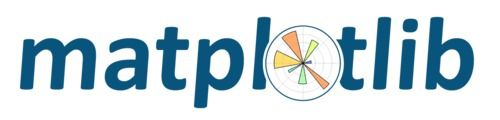Matplotlib is a library for creating informative, attractive, and immersive visualizations in Python. It has several plotting functions for creating line plots, scatter plots, bar plots, error bars, and many other types.

Here are some of the key features and characteristics:

• A range of plot types: There are functions for creating different types of plots, including line plots, scatter plots, bar plots, error bars, and histograms.
• Customization options: It has a comprehensive API for customizing the appearance of plots, such as options for changing colors, fonts, and line styles.
• Support for different backends: It can be used to generate plots in various formats, including PNG, PDF, and SVG, and can be used with different backends like GTK, Qt, and Tkinter, to display plots in different environments.
• Subplots: It has functions for creating subplots that allow you to display multiple plots in the same figure.
• Annotation and text: There are functions for adding text and annotation to plots, such as titles, labels, and legends.

### Seaborn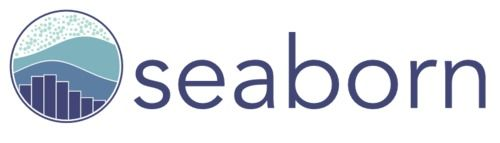Seaborn is used for creating visualizations of statistical data. It is built on top of Matplotlib and provides a high-level interface for creating static, animated, and interactive statistical graphs. It has different functions for visualizing distributions, relationships between variables, and other types of statistical data.

Here are a few features and characteristics:

• Different plot types: It has functions for creating different types of plots, including scatter plots, line plots, bar plots, and distribution plots.
• Customization options: There are options for customizing the appearance of plots like changing colors, styles, and palettes.
• Statistical estimation and plotting: It has functions for estimating and visualizing statistical models like linear regression and kernel density estimation.
• Plotting with categorical data: There are specialized functions for working with categorical data, such as box plots, violin plots, and point plots.
• Plotting with time series data: There are functions for visualizing time series data, such as line plots and kernel density plots.

### scikit-learnscikit-learn is a library for machine learning in Python. It provides algorithms for classification, regression, clustering, and dimensionality reduction as well as tools for evaluating the performance of these models. It also has functions for preprocessing data, such as scaling and imputing missing values.

Some key features and characteristics of scikit-learn are:

• A consistent interface: scikit-learn provides a consistent interface for all of its algorithms which makes it easy to switch between different models and compare their performance.
• Preprocessing and feature engineering: There are functions for preprocessing data and engineering new features, including scaling, normalization, and imputation of missing values.
• Model evaluation: It provides functions for evaluating the performance of machine learning models, such as cross-validation and performance metrics.
• Hyperparameter tuning: It has functions for tuning the hyperparameters of machine learning models like grid search and random search.
• Integration with other libraries: It is compatible with other popular libraries for data manipulation and visualization, such as NumPy, Pandas, and Matplotlib.

### TensorFlow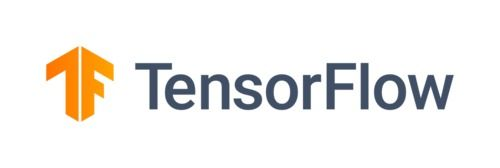TensorFlow is an open-source library for machine learning developed by Google. It is particularly well-suited for deep learning, which is a type of machine learning that involves training artificial neural networks on large amounts of data. TensorFlow provides tools for building and training neural networks along with functions for optimizing their performance.

The key features and characteristics are:

• Numerical computation: TensorFlow provides support for fast and efficient numerical computation using data flow graphs. These allow you to define complex mathematical operations and execute them in parallel which makes TensorFlow suitable for tasks like machine learning and deep learning.
• Machine learning: It has a range of functions and tools for building and training machine learning models, such as support vector machines, decision trees, and neural networks.
• Deep learning: It is ideal for deep learning and has functions for building and training deep learning models. It also offers tools for visualizing and debugging them.
• Hardware acceleration: It can take advantage of hardware acceleration like GPUs and TPUs to speed up the training and inference of machine learning models.
• Cross-platform: It can be used on different platforms, including Windows, macOS, and Linux, and can be used with a variety of programming languages like Python, C++, and Java.

### Keras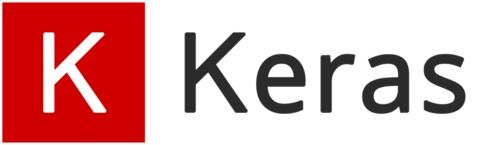Keras makes building and training neural networks faster and easier. It is built on top of TensorFlow and provides a simple, user-friendly interface for defining and training neural networks. It is popular among researchers and practitioners because it allows them to quickly prototype and test new ideas.

Here are some of the key features and characteristics of Keras:

• Model building: Keras offers a simple and intuitive interface for building and training neural networks. It lets users easily define the structure of a network, including the number of layers and the number of units in each layer, and provides a variety of layer types, such as dense, convolutional, and recurrent layers.
• Compilation: Once users have defined a network, they can compile it by specifying the loss function, the optimizer, and any metrics they want to track.
• Training: Keras has functions for training a network on a dataset, including tasks for fitting, evaluating, and predicting.
• Callbacks: It has a flexible callback system that allows users to customize the behavior of their model during training. These include saving checkpoints, early stopping, and custom logging.
• Preprocessing: It has different preprocessing functions and utilities like functions for encoding categorical variables and scaling numerical variables.

### Statsmodels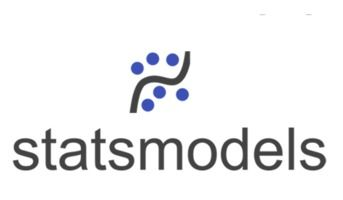Statsmodels is a library for estimating and testing statistical models in Python. It offers functions for fitting models to data and tools for conducting statistical tests and performing hypothesis tests. It is useful for linear regression, time series analysis, and experimental data analysis.

A few features and characteristics:

• Estimation of statistical models: It provides functions for estimating statistical models, including linear, generalized linear, mixed effects, and time series models.
• Testing of statistical hypotheses: It has functions for testing statistical hypotheses like tests of mean, variance, and independence.
• Diagnostic plots: It has functions for creating diagnostic plots like residual, Q-Q, and leverage plots that can be used to assess the fit of a statistical model.
• Model selection: There are functions for selecting the best model from a set of candidate models, such as the Akaike information criterion (AIC) and the Bayesian information criterion (BIC).
• Time series analysis: There is a suite of functions for analyzing time series data, including functions for decomposing time series into trend, seasonality, and residual components, and testing for stationarity and cointegration.

### scikit-image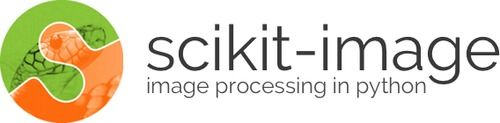As the name suggests, this is a library for image processing in Python. It provides a range of functions for loading, storing, and manipulating images along with algorithms for image segmentation, feature extraction, and feature matching. scikit-image is particularly useful for image classification and object detection.

Some of its key features are:

• Image manipulation: It provides functions for resizing, rotating, and cropping images and functions for adjusting image contrast and brightness.
• Image segmentation: It has functions for partitioning images into regions based on pixel values and other features like color and texture.
• Feature extraction: There are functions for extracting features from images, including edges, corners, and texture patterns.
• Image restoration: There are functions for removing noise and other imperfections from images as well as functions for correcting geometric distortion and perspective.
• Image registration: There are also functions for aligning and registering images which can be useful for combining multiple images into a single composite image or for comparing images taken at different times or from different perspectives.

### PlotlyPlotly is a library for creating interactive visualizations in Python. It has functions for creating line plots, scatter plots, bar plots, and many other types as well as functions for adding interactivity to these plots. With Plotly, you can create plots that can be zoomed in and panned. You can also hover over text displayed, making it a great choice for creating interactive dashboards and data exploration tools.

Here are some of the key features and characteristics:

• Range of plot types: Plotly has functions for creating different types of plots like bar plots, scatter plots, line plots, box plots, and heat maps.
• Customization options: It has customization options for changing the appearance of plots, including options for changing colors, fonts, and styles.
• Interactivity: Plotly plots are highly interactive, allowing users to pan, zoom, and hover to explore the data.
• Web-based visualization: Plotly plots can be easily embedded in web pages, making it easy to share visualizations with others.
• Support for Python and R: Plotly is available for Python and R and has APIs for both languages.

### BokehYou can take visualizations to another level by making them interactive using Bokeh. It is well-suited for visualizing large and complex datasets as it has a fast rendering engine and can handle very large data sizes. Bokeh also has functions for customizing the appearance of plots as well as tools for embedding them in web applications.

### NetworkX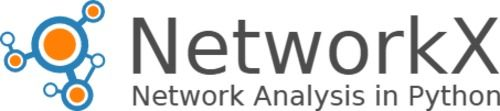NetworkX is a library for working with graph data in Python. It provides data structures for storing and manipulating graphs along with functions for analyzing and visualizing graph data. It is useful for tasks like social network analysis, recommendation systems, and identifying patterns in large and complex datasets.

### PyTorch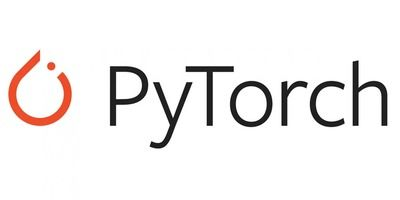Developed by Meta AI, PyTorch is an open-source deep learning library. It is similar to TensorFlow in many ways but has a more dynamic computational graph which makes it easier to debug and modify models during training. PyTorch also has a large community of users and developers, making it a good choice for those who want access to extensive resources and support.

### Beautiful SoupBeautiful Soup is used for parsing and traversing through HTML and XML files. It is useful for web scraping as it can extract data from web pages and convert it into a format that is easy to work with in Python. It is a powerful tool and a must-have for any data scientist working with web data.

### PyPDF2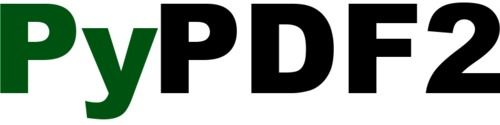PyPDF2 is a library for working with PDF files in Python. It has functions for reading and writing PDFs and tools for extracting and manipulating the data within them. It is ideal for extracting text and images from PDFs, merging and splitting PDFs, and adding annotations and form fields to PDFs.

## Conclusion

There are many Python libraries for data science, each with its own set of features and capabilities. Whether you're working with numerical data, statistical data, or machine learning models, there is a Python library that can help you get the job done. By familiarizing yourself with them and their capabilities, you'll be well-equipped to tackle a range of data science tasks in Python.

## Author

•### Turing

Author is a seasoned writer with a reputation for crafting highly engaging, well-researched, and useful content that is widely read by many of today's skilled programmers and developers.

There are several Python libraries for data science, including Pandas, NumPy, Matplotlib, Seaborn, scikit-learn, SciPy, and Keras.

With over 137,000 libraries, each has unique functions and applications that are important for different aspects of data science. For example, Pandas is used for loading and working with data, NumPy is used for mathematical transformations and calculations, Matplotlib and Seaborn offer various types of visualizations, Keras is a deep learning library, and so on.

Pandas is arguably the most popular data science package since it is equipped with tools and methods to work with all types of data. It has applications in many fields like data analysis, machine learning, deep learning, etc.### Press

What's up with Turing? Get the latest news about us here.### Blog